Download Presentation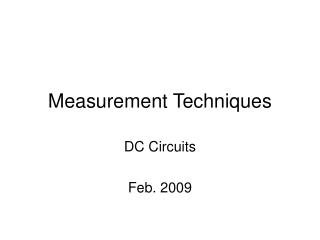Measurement Techniques

# Measurement Techniques - PowerPoint PPT Presentation

Measurement Techniques. DC Circuits Feb. 2009. Measurement Techniques DC Circuits . Resistance (R) Ohms, Ω , K Ω , M Ω Voltage (V) Volt, AC, DC, mV, KV Current (I) Amp, mA (milliamps), uA (microamps). Series Circuit RT = R1 + R2 + R3. R T Ω. R T. R1 R2 R3.I am the owner, or an agent authorized to act on behalf of the owner, of the copyrighted work described.
Download Presentation## Measurement Techniques

An Image/Link below is provided (as is) to download presentation

Download Policy: Content on the Website is provided to you AS IS for your information and personal use and may not be sold / licensed / shared on other websites without getting consent from its author.While downloading, if for some reason you are not able to download a presentation, the publisher may have deleted the file from their server.

- - - - - - - - - - - - - - - - - - - - - - - - - - E N D - - - - - - - - - - - - - - - - - - - - - - - - - -
Presentation Transcript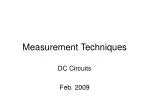### Measurement Techniques

DC Circuits

Feb. 2009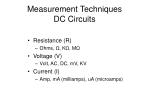Measurement Techniques DC Circuits
• Resistance (R)
• Ohms, Ω, KΩ, MΩ
• Voltage (V)
• Volt, AC, DC, mV, KV
• Current (I)
• Amp, mA (milliamps), uA (microamps)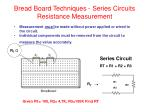Series Circuit

RT = R1 + R2 + R3

RTΩ

RT

R1

R2

R3

• Measurement must be made without power applied or wired to the circuit.
• Individual components must be removed from the circuit to measure the value accurately.

Given R1= 100, R2= 4.7K, R3=100K Find RT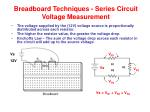Vs

12V

VR1

R1

R2

R3

Vs

VR2

VR3

Vs = VR1 + VR2 + VR3

Breadboard Techniques - Series Circuit Voltage Measurement
• The voltage supplied by the (12V) voltage source is proportionally distributed across each resistor.
• The higher the resistor value, the greater the voltage drop
• Kirchoffs Law – The sum of the voltage drop across each resistor in the circuit will add up to the source voltage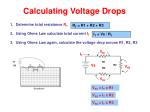Vs

12V

IT

VR1

R1

R2

R3

Vs

VR2

VR3

VR1 = IT x R1

VR2 = IT x R2

VR3 = IT x R3

Calculating Voltage Drops

RT = R1 + R2 + R3

• Determine total resistance RT
• Using Ohms Law calculate total current IT
• Using Ohms Law again, calculate the voltage drop across R1, R2, R3

IT = Vs / RT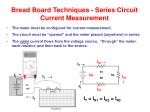IR1

IT

R1

R2

R3

Vs

Vs

12V

IR2

IT

IT

IT

IR3

IT = IR1 = IR2 = IR3

IT

Bread Board Techniques - Series Circuit Current Measurement
• The meter must be configured for current measurement.
• The circuit must be “opened” and the meter placed (anywhere) in series.
• The same current flows from the voltage source, “through” the meter, each resistor, and then back to the source.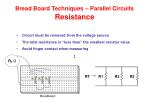RTΩ

RT

R1 R2 R3

Bread Board Techniques – Parallel CircuitsResistance
• Circuit must be removed from the voltage source
• The total resistance is “less than” the smallest resistor value
• Avoid finger contact when measuring

1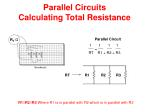1 1 1 1

RTΩ

Parallel Circuit

RT R1 + R2 + R3

=

RT

R1 R2 R3

Parallel CircuitsCalculating Total Resistance

R1//R2//R3 Where R1 is in parallel with R2 which is in parallel with R3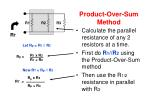Let Rp = R1 // R2

R1 x R2

R1 + R2

Rp =

R1 R2 R3

Now RT = Rp // R3

RT

Rp x R3

Rp + R3

RT =

Product-Over-Sum Method
• Calculate the parallel resistance of any 2 resistors at a time.
• First do R1//R2 using the Product-Over-Sum method
• Then use the R1/2 resistance in parallel with R3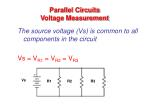R1 R2 R3

Parallel Circuits Voltage Measurement

The source voltage (Vs) is common to all components in the circuit

Vs = VR1 = VR2 = VR3

VsI1

I2

I3

IT

Parallel Circuits Current Measurement

I1 + I2 + I3

Vs

R1

R2

R3

I2 + I3

IT= I1 + I2+ I3IT

I1

I2

I3

Vs

R1

R 2

R3

Parallel CircuitsCurrent Calculations

To measure current the circuit must be broken and the current meter must be placed in series with the component.Vs

50V

R1

150 Ω

R2

300 Ω

R3

100 Ω

Calculating Total Current (IT)
• First find total resistance RT

2. Then use Ohm’s Law to find total current

Using Product-Over-Sum Method

R1//R2 = (150 x 300) / (150 + 300) = 100 ohms

Rp//R3 = (100 x 100) / (100 + 100) = 50 ohms

Note: when the parallel resistors are equal in value, just divide by the number of R’s

3K//3K = 1.5K

6K//6K//6K = 2K

Using Reciprocal Method

1/RT = 1/R1 + 1/R2 + 1/R3 = 1/150 + 1/300 + 1/100

= 0.00666 + 0.00333 + 0.01 = 0.020

RT = 1/ 0.020 = 50 ohmsVs

50V

R1

150 Ω

R2

300 Ω

R3

100 Ω

Total Current IT

Vs

RT

IT =

Calculating Total Current (IT)
• First find total resistance RT

2. Then use Ohm’s Law to find total current

50 v

= -------- = 1 amp

50 Ω

The power supply must be capable of supplying at least 1 amp of currentCalculating Branch Currents

RT = 50 ohms IT = 1 amp

IT

I1

I2

I3

Vs

50 V

R1=150

R2=300

R3=100

I1 = Vs / R1 = 50/150 = 0.333333 amps

I2 = Vs / R2 = 50/300 = 0.166666 amps

I3 = Vs / R3 = 50/100 = 0.200000 amps

1.00 ampSeries/Parallel Circuits
• There are multiple current paths.
• Resistors may be in series or parallel with other resistors.
• A node is where three or more paths come together.
• The total power is the sum of the resistors’ power.--/\/\/\/\--

Rs

I

R

E

Simple Combo circuit

Reduce the parallel connection to its series equivalent

R2 // R3 = Rs

Then reduce the series equivalent to the total resistance as seen by the source

RT = R1 + RsR1 R3

R2 R4

R1 R3

+ +

R2 R4

RT = R1,2 // R3,4

Reduce & Simplify100 200

200 400

12 V

Analysis of a combo circuit
• Calculate
• Total current
• Branch currents
• IR drops

Board Solution100 200

200 400

300 600

12 V

12 V 200 Ώ

Reduce & Simplify – find RT

RT = R1,2 // R3,4

= 300 // 600 = 200

IT = 12 / 200 = 0.06 amps (60 mA)R1 R2 R3

R1 R2 R3

RT

IT

Determining Total Resistance

1 1 1 1

RT = R1 + R2 + R3

V

RT

RT = V

IT100 200

200 400

300 600

12 V

Branch Currents

IT Ia Ib

Branch Currents

Ia = 12 / 300 = 40 mA

Ib = 12 / 600 = 20 mA

IT = Ia + Ib = 40mA + 20 mA = 60 mA60 mA 40 mA 20 mA

R1 R3

100 200

R2 R4

200 400

12 V

IR Drops (voltage across each resistor)

VR1 = 40 mA x 100 = 4000 mV = 4V

VR2 = 40 mA x 200 = 8000 mV = 8V

VR3 = 20 mA x 200 = 4000 mV = 4V

VR4 = 20 mA x 400 = 8000 mV = 8VVA = R2 x Vs

R2 + R1

VB = R4 x Vs

R4 + R3

R1 R3

R2 R4

AB

Vs

VAB

VA

VB

Bridge Circuit

In a bridge circuit the voltage difference between the two parallel branches is used to indicate the potential difference between the two points.

VAB = VA - VB

Using the Voltage Divider FormulaWheatstone Bridge – null balance detector

VOUT = 0 volts

A balanced bridge can be used to measure an unknown resistance.

The Wheatstone bridge can be used as an “ohmmeter” by comparing the unknown resistance value to a known one.R1

R1

VOUT

A

B

Vs

R1

Rs

Conditioning circuit for resistive sensors and transducers
• VOUT can be used to represent some type of process variable
• Temperature
• Thermistor
• Resistance Temperature Detectors (RTD’s)
• Pressure
• Strain Gauge
• Flow
• Anemometer

The bridge is often used as a conditioning circuit to convert the output of aresistivetype sensing element into a voltage (mV)